# Physics and Instrumentation for Duplex Scanning

Physics and Instrumentation for Duplex Scanning

Daniel F. Leotta

Kirk W. Beach

Ultrasonic duplex scanning, the combination of two-dimensional (2D) ultrasound B-mode imaging and single-gate pulse Doppler blood velocimetry with spectral waveform analysis, is the most successful and widely used noninvasive method available for the examination and classification of vascular disease. This chapter focuses on the physics and instrumentation behind these ultrasound methods for the examination of blood vessels. This is not a comprehensive reference, but rather a review of selected topics in order to emphasize their importance or to offer a different perspective from those found in standard physics texts.

ULTRASOUND PROPAGATION THROUGH TISSUE

Linear Mechanics of Longitudinal Waves

The basis of all ultrasound imaging methods is the propagation of sound waves through tissue. Sound waves are mechanical waves. Two common types of mechanical waves are transverse waves and longitudinal waves. For a transverse wave, particle motion is perpendicular to the direction of wave propagation. For a longitudinal wave, particle motion is parallel to the direction of wave propagation. In tissue, transverse waves are attenuated in short distances, converting all of the wave energy into heat. However, ultrasound does not travel through tissue as a transverse wave; it passes through tissue as a longitudinal wave, with regions of tissue compression and regions of tissue decompression. The pressure increase and decrease as an ultrasound wave passes can be several times the atmospheric pressure. Although the motion of the tissue as the ultrasound wave passes is just a few nanometers, the maximum molecular velocities are near 1 cm/s, and the accelerations are nearly 1000 times the acceleration of gravity.

The physical principles of ultrasound transmission can be derived from Newton’s equations using a model of masses (representing molecules) and springs (representing chemical bonds) (Fig. 7.1):

Force = mass × acceleration, and Force = stiffness × compression

When these equations are applied to molecules in a material, the compression force is set equal to the acceleration force:

Molecular force = density × molecular acceleration = stiffness × (compression change with distance)

Ultrasound wave mechanics are derived from this equation. If u is the distance of each molecule from its resting position, y is distance along the direction that the wave is traveling, and t is time, the previous equations become

Molecular force = density × d2u/dt2 = stiffness × d2u/dy2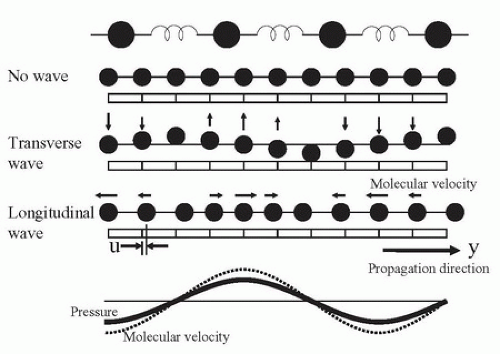FIGURE 7.1. Mass and spring model for tissue mechanics. The ultrasound transmission properties of tissue can be derived by considering the tissue to be assembled from masses (representing tissue density) and springs (representing tissue stiffness). No wave: At rest, with no wave present, the masses are equally distributed in the tissue. Transverse wave: If a transverse wave passes through the tissue, the displacement of the tissues can be seen, like the lateral displacement as a wave passes along a rope. Longitudinal wave: Ultrasound passes through tissue as a longitudinal wave, with regions of tissue compression and regions of tissue decompression. Pressure fluctuation and molecular velocity: The pressure fluctuation and the molecular velocity are in phase; the ratio of pressure fluctuation to molecular velocity is the tissue impedance. The acceleration is ¼ cycle ahead of the molecular velocity and the displacement is ¼ cycle delayed.

where the second derivatives d2u/dt2 and d2u/dy2 represent tissue acceleration and tissue distortion, respectively. These equations can be solved by assuming that molecular displacement u is dependent on the variable group (y + C × t) having units of centimeters; C has units of centimeters per second (cm/s). The previous derivatives can then be expressed as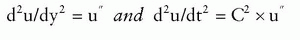and the equation becomes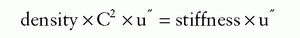Therefore, for any waveshape of u, the equation is correct if C2 = (stiffness/density). In this equation, C2 is positive, but the value of C can be either positive or negative, and it is the speed of the ultrasound wave.

The meaning of C is further understood by looking at Figure 7.2. The wave has different locations at different times. As time advances (from top to bottom), the wave moves from left to right. The line marked “wave speed” shows a place on the wave near a crest where the value (y + C × t) is constant. In Figure 7.2, C is negative; thus, as time increases, y must also increase to keep (y + C × t) constant. The expression (y + C × t) provides the relationship between advancing time and advancing location, which is speed. The wave period T (the time it takes to change from one peak to the next) and the wavelength λ (the distance it takes to change from one peak to the next) are related by C, the wave speed:

C = (wave length)/(wave period) = λ/T

Wave period (T) is the inverse of frequency (F), so that

C = λ × F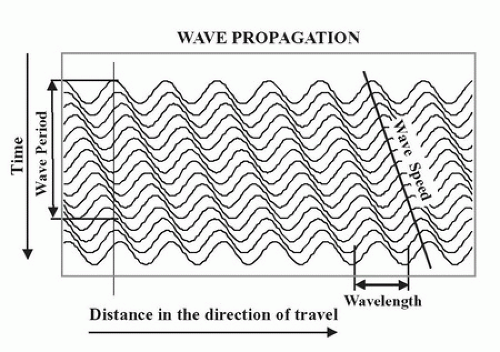FIGURE 7.2. Wave propagation over time. As a wave travels through a medium, the wave length (λ) and the wave period (T = 1/F) are related by the wave speed (C = λ/T = λ × F). The wave function is dependent on the variable (y – C × t) where y is the distance along the direction of travel and t is time.

The following equation is also an important relationship to remember for any longitudinal mechanical (sound) wave traveling through a material: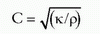where κ is stiffness and ρ is density. Of course, both stiffness and density depend on temperature; thus, C will vary with temperature.

The speed of sound in typical soft tissue is 1540 m/s or 1.54 mm/µs, whereas the speed of sound in air is 331 m/s. These are determined by the density and stiffness of the materials. Typical sound and ultrasound wavelengths are presented in Table 7.1.

Ultrasound Frequencies and Wavelength

Frequency is measured in hertz, which is an expression of cycles of compression and decompression per second. Medical ultrasound frequencies are measured in millions of cycles per second, or megahertz (MHz). Wavelength is related to the spatial resolution of an ultrasound image. Because your ears are about 15 cm apart, you cannot tell where a 20-Hz sound (15-m wavelength in air) is coming from, but you can identify where a 2-kHz sound (15-cm wavelength in air) is coming from. By using 5-MHz ultrasound in tissue (0.3-mm wavelength), it is possible to resolve objects that are a few millimeters apart.

TABLE 7.1 SOUND WAVELENGTHS FOR DIFFERENT FREQUENCIES

 ▪ SOUND FREQUENCY ▪ WAVELENGTH IN AIR (FOR C = 300 m/s) ▪ WAVELENGTH IN TISSUE (FOR C = 1500 m/s) 20 Hz 15 m 75 m 1 kHz 30 cm 1.5 m 20 kHz 15 mm 7.5 cm 1 MHz 0.3 mm 1.5 mm 10 MHz 0.03 mm 0.15 mm C, wave speed (see text).

Impedance and Wave Speed

As a sound pulse (or wave) travels through tissue, zones of high pressure and low pressure are created. The high-pressure regions occur where the molecules are squeezed together, and the low-pressure regions occur where the molecules are spread apart. The pressure elevation or depression from atmospheric pressure is called pressure fluctuation (p). Pressure fluctuation is equal to the stiffness (κ) times du/dy. As a sound pulse travels through tissue, the molecules oscillate in the direction of the sound wave. The instantaneous molecular velocity (v) of the oscillating molecules is du/dt, and because u is dependent on (y + C × t), du/dt = C × du/dy. Therefore, v/C = p/κ, or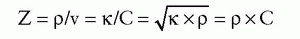Z is called the acoustic impedance of the tissue. In physical terms, impedance is the ratio between the pressure fluctuation that the tissue feels as a wave passes and the molecular velocity of the molecules as the wave passes. Be sure to avoid confusing the molecular velocity of the molecules (v) with the wave speed (C). The molecular velocity (v) oscillates in the positive and negative directions during ultrasound wave passage at the ultrasound frequency; v is greater when the wave intensity is greater. C is the speed at which the wave passes and does not change with wave intensity, as long as the intensity is low. If the intensity is high, the “linear” model of springs and masses in Figure 7.1 does not hold. The “nonlinear” waves at higher intensities cause harmonics, which are the basis of harmonic imaging (discussed later in this chapter).

It is important to remember that impedance (Z), like wave speed (C), is dependent on the density and the stiffness (1/elasticity) of the tissue. Just as wave speed changes with temperature, impedance changes with temperature because temperature affects both stiffness and density.

Amplitude and Phase

In addition to the wavelength (in units of distance) and period (in units of time), each wave has two other properties: the amplitude and the phase (Fig. 7.3). All of the information in a wave is encoded in the amplitude and phase. Ultrasound B-mode imaging displays the echo amplitude, and Doppler velocity information is acquired from the phase. These two kinds of information are independent: one can change while the other remains constant.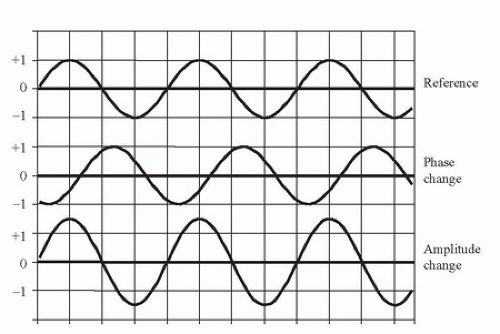FIGURE 7.3. Wave phase and amplitude. Two parameters define a wave of a particular frequency: the amplitude and the phase. The amplitude of a wave is taken as the peak difference of a parameter from ambient conditions. In ultrasound, the parameter is usually pressure fluctuation from atmospheric pressure, but could be molecular velocity, acceleration, or displacement. Amplitude of the ultrasonic echo is used for B-mode imaging. The phase is the time difference of a wave feature from the same feature on a reference wave. Therefore, phase is always represented as a difference. That difference could be in time or in distance along the propagation direction.

The amplitude of a sound wave can be measured in many different ways, but each method measures properties experienced by the molecules of the material through which the sound is traveling. Each molecule experiences a pressure fluctuation, which is a displacement back and forth along the direction of wave travel associated with a molecular velocity and acceleration. The displacement, velocity, and acceleration are like the displacement, velocity, and acceleration of a swing or a pendulum.

Frequency and phase are closely related. When a 5-MHz Doppler looks at an approaching blood velocity of 75 cm/s, the Doppler echo has a frequency of 5.005 MHz. The frequency is increased by 0.1% or 1/1000 of the transmit frequency. Another way to think of this is that the phase becomes more advanced with every cycle: for every 1000 cycles, the phase has advanced 1 cycle. After the first 10 cycles, the phase has advanced 1/100 of a cycle. Because one cycle is 360 degrees, after 10 cycles, the phase has advanced 3.6 degrees, and after 100 cycles, the phase has advanced 36 degrees. It can be convenient to think about the Doppler shift as a continuing change in phase rather than a frequency shift.

Pressure Fluctuation and Molecular Velocity, Displacement, and Acceleration

Molecular velocity, displacement, acceleration, and pressure are related to ultrasound intensity. These four measured values are ways to look at the mechanical “shaking” that the molecules experience as an ultrasound wave passes through the tissue. First, ultrasound intensity is related to energy:

Energy = force × distance

Power = energy/time = force × distance/time

Intensity = power/area = force/area × distance/time = pressure fluctuation × molecular velocity

As discussed earlier, the pressure fluctuation is the fluctuation of the pressure in the tissue due to the passing of an ultrasound wave; the molecular velocity is the velocity of molecules in the tissue due to the passing of the ultrasound wave.

As shown previously, in the solution to Newton’s equations, the ratio of tissue pressure fluctuation to molecular velocity fluctuation represents the tissue impedance (Z):

Z = (pressure fluctuation)/(molecular velocity)

By substitution:

Intensity = (pressure fluctuation)2/Z = Z × (molecular velocity)2

If the ultrasound is continuous wave (CW), the average intensity is the average of the sine wave amplitude squared. That average is half of the squared maximum value:

Average intensity = (maximum pressure fluctuation)2/2 × Z = Z/2 × (molecular velocity)2

This is also true within an ultrasound pulse. The temporal peak intensity is computed from this same expression.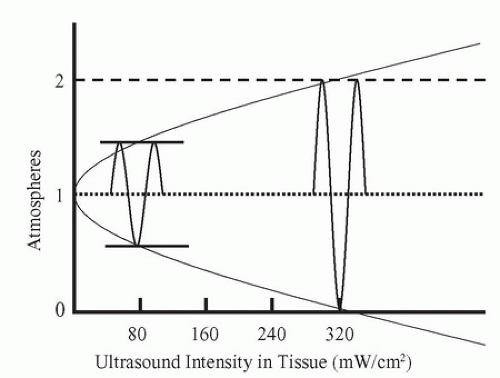FIGURE 7.4. Tissue pressure fluctuation versus medical ultrasound intensity. Intensity is equal to one-half of the square of the peak pressure fluctuation divided by the tissue impedance. Using the impedance of water, which is near the impedance of human tissues, at an intensity of 320 mW/cm2 (SPTP), the pressure fluctuation equals 1 atm.

A graph of the pressure fluctuations versus CW intensity (Fig. 7.4) indicates that a physical problem occurs within the range of diagnostic ultrasound intensities: at temporal peak intensities greater than 320 mW/cm2, the pressure fluctuation becomes greater than 1 atm. Therefore, the minimum pressure theoretically becomes negative. This is impossible according to the physical laws of thermodynamics. Thus, the linear equation for compressibility, which is based on thermodynamics, does not apply for these conditions. The wave becomes nonlinear (Fig. 7.5) and gives rise to harmonics.

Nonlinear Mechanics

With nonlinear mechanics, the linear equations presented earlier no longer apply. The relationship between pressure and displacement, which is based on the nature of molecular bonds, does not hold for large fluctuations in pressure.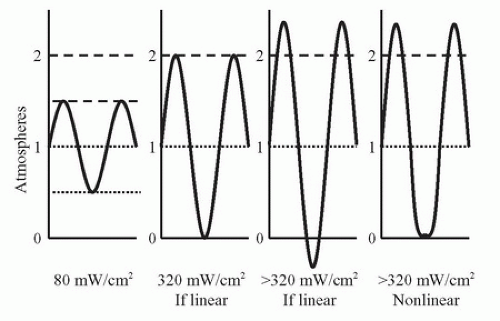FIGURE 7.5. Ultrasound waveshape changes with nonlinear propagation. As an ultrasound sine wave propagates through tissue, the shape of the wave changes: the peaks increase in height and sharpness, and the valleys become blunted. This effect is greater at higher intensities. This change in shape is due to nonlinear stiffness of the tissue, which causes the wave velocity to be higher when the pressure is at the peaks and the wave velocity to be lower when the minimum pressure is at or below zero.

The nonlinear relationship between compression and force is shown in Figure 7.6. In the central region, stiffness is linear, but when compression becomes too great or too small, the effective stiffness changes. When the wave intensity is very large, during compression, the stiffness is increased, causing an increase in the wave speed and an increase in the wave impedance. Likewise, during decompression, the stiffness is decreased, causing decreases in both the wave “speed” and “impedance.” Speed and impedance are in quotation marks in these cases because their definitions become less useful in these nonlinear conditions than in the linear conditions.

Harmonics and Ultrasound Contrast Agents

The flattened valleys and the enhanced peaks of the waveshape in Figure 7.5 (right) can be represented as a combination of sine waves (Fig. 7.7). In this example, a sine wave at the original frequency and a sine wave at two times the original frequency are shown. The wave at the original frequency is called the fundamental; the wave at two times the frequency is called a harmonic. The wave at two times the fundamental frequency is called by different names: it is called the “first harmonic” by some people and the “second harmonic” by others. Both groups agree on the name “first overtone” for that frequency. Harmonics occur at two times, three times, or any integer multiple of the fundamental. Any periodic (repeating) waveshape can be formed from a series of sine wave harmonics of the fundamental by selecting the phase and the amplitude of each harmonic. This is the Fourier theorem and is the basis of the Fourier transform. The lower curve in Figure 7.7 shows the combination of the fundamental and the harmonic.

Harmonics are present in all diagnostic ultrasound echoes. If the transmitted intensity is increased, the intensity of the harmonics increases as more of the power from the fundamental frequency is converted to the harmonics. The attenuation of ultrasound is proportional to frequency; thus, the harmonics are attenuated more rapidly than the fundamental. Some of that attenuation is due to absorption (conversion to heat), so that if the ultrasound intensity is doubled, the amount of ultrasound converted to heat more than doubles because of the conversion to harmonics and the subsequent conversion to heat.

The subject of harmonics has been recognized for decades but was not often discussed until recently, and harmonic displays are relatively recent additions to diagnostic ultrasound instruments. Interest in the display of harmonics is primarily a result of a general desire to improve detection of ultrasound contrast agents. When ultrasound contrast agents were introduced, they were designed to increase the strength of the echo signals in ultrasound images. However, they did not produce the strong echoes on images that were hoped for. This led to the development of methods to display harmonics. The bubbles in ultrasound contrast agents will change the linear portions of the line in Figure 7.6 and cause the generation of harmonics. Thus, displaying harmonic echoes was expected to show contrast agents more prominently. However, tissues without contrast agents also reflect harmonic echoes back to the transducer (Fig. 7.8). In general, tissue harmonic imaging improves the lateral resolution and image contrast.

To generate a harmonic image using a 3-MHz transducer, it is logical to transmit at 3 MHz and receive at 6 MHz, but this cannot be done because a transducer is not sensitive at even multiples of the frequency. The 3-MHz transducer must have a “damping” material to make it “broadband,” so that it will operate between 1.5 and 4.5 MHz. Then, for harmonic imaging, it is possible to reduce the transmit frequency to 2 MHz and increase the receive frequency to select 4-MHz echoes to generate the harmonic image.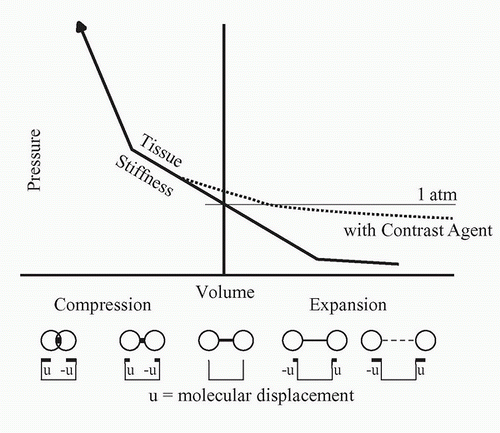FIGURE 7.6. Nonlinear behavior of tissue stiffness. Near atmospheric pressure, the compressional force is linearly related to the change in dimension of the tissue, but at higher or lower pressures, the force significantly deviates from linear. The addition of an acoustic contrast agent consisting of bubbles expands the tissue and increases the nonlinearity.

Transmitted Ultrasound

Continuous Wave

CW ultrasound is used for Doppler applications. The instruments are generally inexpensive and often provide nondirectional audible output. However, CW Doppler instruments can provide directional information, and they can also produce spectral waveforms. The transmitted ultrasound is “narrowband” because only one ultrasound frequency is transmitted. Because the transmission is continuous, no information about the depth of the detected flow is available.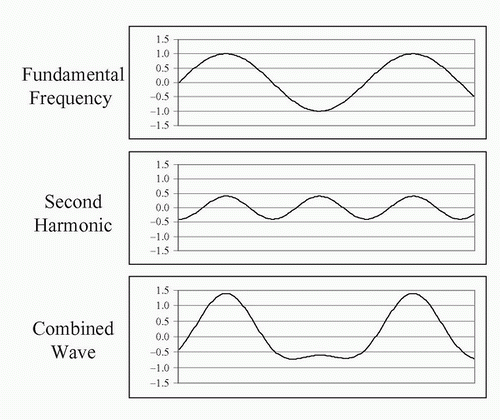FIGURE 7.7. Harmonic components of nonsinusoidal waves. Any periodic repeating wave can be represented by a series of sine waves starting with the fundamental and adding wave frequencies that are multiples of the fundamental. These waves are harmonics. The first harmonic is the fundamental, the second harmonic has a frequency twice that of the fundamental, and so on. Each harmonic has a unique amplitude and phase that it contributes to the combined wave. Here, 1.5 cycles of the fundamental (top) are added to 3 cycles of the second harmonic (middle) with a phase that causes the peaks to align, producing a wave with blunted minimums and enhanced maximums (bottom). The combined wave is similar to the wave in Figure 7.5 that resulted from nonlinear propagation in tissue.

Burst and Pulse

The terms pulse and burst have similar meanings. Pulse refers to the shortest burst of ultrasound that can be sent into tissue with the transducer available. Burst refers to an intentionally prolonged transmit oscillation. For most diagnostic imaging applications, the transmitted ultrasound pulse is on for a short period of time (Fig. 7.9), less than a microsecond, and off for 100 µs. For most pulsed Doppler applications, a burst of ultrasound lasting 1 µs is sent into tissue. For transcranial Doppler applications, the transmitted burst may last 15 µs. Long transmit bursts are narrowband, so they define the Doppler frequency with precision and they are resistant to noise. For B-mode imaging, a short (broadband) transmit pulse is used to ensure the best
(smallest) depth resolution. This allows the visualization of small structures in the depth direction, like the intima-media thickness.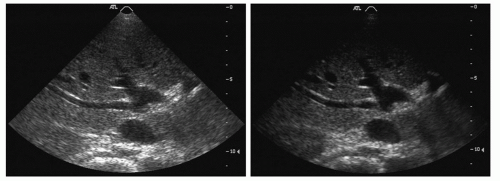FIGURE 7.8. Harmonic image of liver without contrast agent. In typical medical ultrasound imaging, the SPTP intensities are much higher than 320 mW/cm2, so nonlinear wave propagation and the formation of harmonics are common. An image formed by showing the amplitude of the fundamental frequency echo (left) looks different from an image of the same tissue formed by transmitting a lower frequency and forming an image based on the strength of the second harmonic of the transmitted frequency (right).

During the “off” period, the receiver is accepting echoes from successively deeper locations. This period lasts between 40 and 400 µs. An ultrasound instrument computes the depth of the echo reflector by measuring the time from the transmit pulse to the echo, assuming an ultrasound speed of 1.54 mm/µs. Echoes from shallow structures (1 cm deep) return soon after the transmit pulse (about 13 µs); echoes from deeper structures (3 cm deep) return later after the transmit pulse (about 40 µs); echoes from the deepest structures (25 cm deep) return latest (about 325 µs) (Table 7.2).

Duty Factor

The duty factor (DF; or duty cycle) is a measure of the fraction of time that the ultrasound instrument is transmitting. In a CW instrument, the system is always transmitting, and the DF is 1.0, or 100%. In a pulse-echo B-mode ultrasound instrument imaging a maximum depth of 19 cm, the transmit pulse is 1 µs, and the pulse repetition period (PRP; time between pulses) is 250 µs. Thus, the DF is 1/250, or 0.004, or 0.4%. The concept of DF also applies to a home heating system. To heat a home on a cool day, the furnace might be on for 15 minutes out of every hour (DF = 25%), but on a cold day, the furnace might be on for 30 minutes out of every hour (DF = 50%). The DF is an important part of the computation of ultrasound intensities.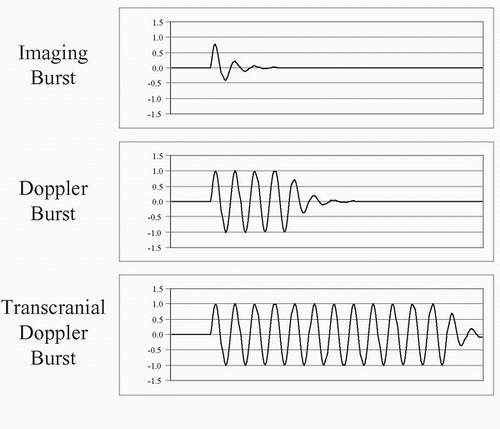FIGURE 7.9. Burst length of ultrasound pulses. For B-mode imaging, short (broadband) ultrasound bursts are transmitted into tissue (top) to get the best depth resolution. For Doppler, medium-length ultrasound bursts are transmitted into tissue (middle). For transcranial Doppler, in which the echoes are greatly attenuated and the transmitted burst energy must be high while limiting the SPTP intensity, long (narrowband) ultrasound bursts are transmitted into tissue (bottom).

Power and Intensity

There is a great deal of confusion in ultrasound physics literature about power and intensity. Here is the reason for the confusion. Power is a measure of energy per time and has units of watts. As an ultrasonic wave passes through tissue, the power
is distributed over the cross-sectional area of the ultrasound beam pattern. Power divided by the cross-sectional area is equal to the intensity. The intensity is easily measured with an ultrasound transducer called a hydrophone and determines whether the ultrasound propagation is linear or nonlinear. Intensity is related to pressure fluctuation and is therefore the quantity usually discussed in ultrasound physics. Intensity is dependent on both the ultrasound power in the beam pattern and the cross-sectional area of the beam pattern. Changes in intensity due to changes in cross-sectional area of the beam pattern can easily be confused with changes in intensity due to changes in ultrasound power. It is important to keep the two factors separate.

TABLE 7.2 TIME REQUIRED FOR AN ECHO TO RETURN FROM A KNOWN DEPTH

 ▪ DEPTH (cm) ▪ TIME (MINIMUM POSSIBLE PRI) (µs) ▪ MAXIMUM POSSIBLE PRF (kHz) 1 13 60 2 26 40 3 39 20 5 65 12 10 130 6 15 195 5 20 260 4 25 325 3 PRF, pulse repetition frequency; PRI, pulse repetition interval.

In tissue, ultrasound power decreases with distance as the wave propagates. The decrease in power is due to attenuation in the tissue. Attenuation has two factors: (1) conversion of the ultrasound power to heat (absorption) and (2) scattering of the ultrasound power in directions other than the direction of the ultrasound beam. The attenuation (both absorption and scattering) of ultrasound in tissue is dependent on the tissue type and the ultrasound frequency.

Measures of Ultrasound Intensity

There are six common measures of ultrasound intensity:

• Spatial average temporal average (SATA)

• Spatial peak temporal average (SPTA)

• Spatial average pulse average (SAPA)

• Spatial peak pulse average (SPPA)

• Spatial average temporal peak (SATP)

• Spatial peak temporal peak (SPTP)

Between 1980 and 1990, the number of measures was changed from four to six, and the naming of the measures changed. The new measures are pulse average values, which were previously called “temporal peak.” Temporal peak now refers to an instantaneous peak value rather than a value averaged over the pulse. Two of the six measures (SATA and SPTA) are used for computing heating effects; the rest are used for considering ultrasonic cavitation and nonlinear effects of ultrasound.

All of these are measures of an ultrasound transmit beam and depend on two factors: (1) the beam power and (2) the beam area. The initial power of the beam is selected by applying the proper voltage to the ultrasound transducer based on the transducer thickness, the damping material on the back of the transducer, the area of the face of the transducer, and the efficiency of coupling to the body tissues under examination:

Initial beam power = (transducer voltage)2 × (transducer area) × (coupling to tissue)/damping

As the ultrasound pulse proceeds into tissue, the beam power decreases owing to attenuation:

Beam power = initial beam power × (attenuation rate)depth

The beam area is dependent on the focal character of the transducer. Intensity is the ratio of beam power to beam area:

Intensity = beam power/beam area = W/cm2

The beam power can be expressed as a maximum or as a temporal average.

The most widely accepted method of measuring the ultrasound beam is to begin with a measurement of the total beam power. Beam power is measured by directing the beam onto a submerged weighing pan of a standard balance. When the ultrasound beam strikes the pan, a force appears. The force is equal to

Force = 2 × power/C

In water, where the speed of sound is 148,000 cm/s, a 1-W temporal average ultrasound beam generates a force equal to the weight of a 1.38-mg mass. This force can be demonstrated by imaging water in a tank. By turning up the gain, particles suspended in the water can be seen. As the transmit power is increased, the suspended particles can be seen on the ultrasound image rushing away from the ultrasound scanhead because of the ultrasound force. By measuring the ultrasound beam with a hydrophone, the beam diameter and area can be determined. From these measurements, the SATA intensity can be determined:

SATA = power/area

A look at the interrelationships of the six intensity terms listed above can provide a more complete picture of tissue exposure. The intensity measures are related by combinations of four factors: (1) duty factor (Fig. 7.10), (2) beam factor (Fig. 7.11), (3) pulse factor (Fig. 7.12), and (4) image factor (Fig. 7.13). These four factors all have the same range: maximum = 1, minimum = 0. Using a hydrophone and an oscilloscope (which traces the voltage in time that is generated by the hydrophone),
the factors shown in Figures 7.10,7.11,7.12 and 7.13 can be determined. Then, the intensities can be computed: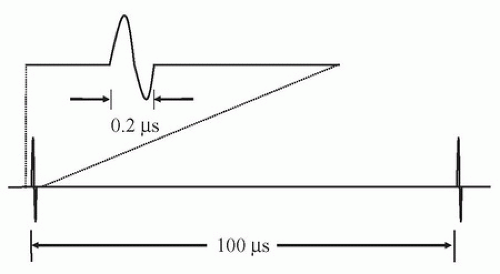FIGURE 7.10. Duty factor. The duration of an ultrasound transmit burst (top) is short compared with the time interval separating bursts (bottom). The ratio of burst length to interval is called the duty factor.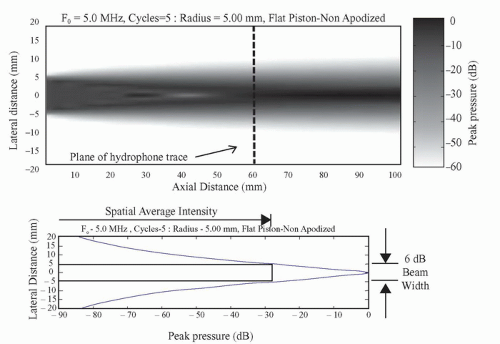FIGURE 7.11. Beam factor. Top, The beam pattern of a flat circular transducer extends from the face of the transducer into tissue. Near the transducer in the Fresnel zone is a complex area of varying intensity formed by diffraction, which is the constructive and destructive interference of the waves from the transducer face. Far from the transducer in the Fraunhofer zone, the beam pattern becomes much smoother. Bottom, A profile of the maximum pressure fluctuation taken across the beam at the transition zone between the Fresnel and the Fraunhofer zones shows the intensity peak. A box is drawn that shows the width of the central portions of the beam where intensities are at least 25% (>6 dB) of the peak. The spatial average intensity across that beam width is between one-half and one-third of the central peak intensity.

SPTA = SATA/beam factor

SAPA = SATA/DF

SATP = SATA/DF/pulse factor

SPPA = SATA/beam factor/DF

SPTP = SATA/beam factor/DF/pulse factor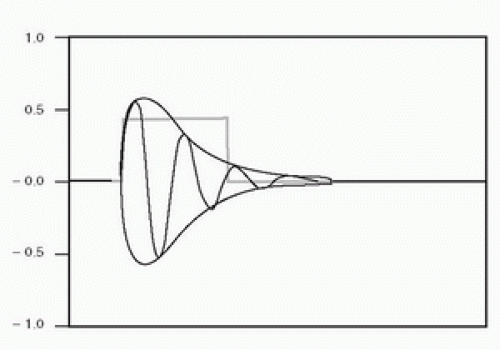FIGURE 7.12. Pulse factor. The oscillations show the acoustic pressure applied to the tissue, with a gradual onset and decay. During each half-cycle, the energy is proportional to the square of the amplitude, indicated by the envelope of the pulse. The square pulse is a simplified model of the ultrasound burst power showing the temporal average power for the duration of the pulse. The pulse average power is less than the instantaneous temporal peak power of the pulse.

Different ultrasound modes have different DFs (Table 7.3). In some examinations, the ultrasound beam is held stationary (A-mode, M-mode, and pulsed Doppler). For 2D imaging, however, the scanhead sweeps the ultrasound beam across a plane of tissue, penetrating each element of tissue only once per image frame for 2D B-mode, and typically eight times
per image for 2D color Doppler. Thus, in 2D imaging, the image factor must be included in exposure computations for a voxel (a small volume) of tissue, indicating the number of ultrasound scan lines per image that are acquired from other locations in tissue. This allows an increase in the energy in each transmit burst, because only one ultrasound pulse passes through each voxel of tissue in each frame. The frame rate is usually about 30 images/s; thus, only 30 pulses/s heat each segment of tissue. However, because the bursts have higher energy, they have high peak positive and negative pressures, which thereby increase the chance of cavitation in the tissue.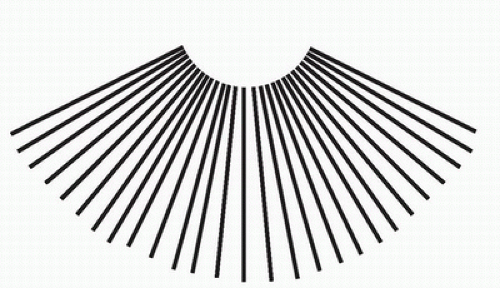FIGURE 7.13. Image factor. The 2D B-mode image is formed from more than 100 pulse-echo lines. Therefore, the average intensity exposure of tissue on any one line is equal to the exposure computed for repeat exposures if all pulses were delivered along a single line, divided by the number of lines in the image.

TABLE 7.3 TRANSMIT PARAMETERS FOR ULTRASOUND EXAMINATION METHODS

 ▪ METHOD ▪ F (MHz) ▪ IF ▪ PRF (kHz) ▪ PRP (µs) ▪ PD (µs) ▪ DF Continuous-wave Doppler 5 1 — — — 1.00 Transcranial pulsed Doppler 2 1 5 200 10 0.05 Cardiovascular pulsed Doppler 5 1 10 100 1 0.01 M-mode imaging 5 1 1 1000 0.5 0.0005 2D B-mode imaging 5 0.01 5 200 0.5 0.000025 2D color-flow imaging 5 0.05 5 200 1 0.00025 DF, duty factor (dimensionless); F, ultrasound frequency; IF, image factor = 1/number of ultrasound lines in an image (dimensionless); PD, pulse duration = length of transmit pulse or burst (s); PRF, pulse repetition frequency = number of transmitted pulses per second (Hz); PRP, pulse repetition period = 1/PRF (s); 2D, two-dimensional.

Theoretical Intensities versus Actual Intensities

Unknown factors, such as the attenuation of overlying tissue and refractive spreading of the ultrasound beam, make correct theoretical computations of the ultrasound intensities impossible. Experimental investigations of ultrasound intensities must be performed if accurate values are to be known. Unfortunately, properly mimicking the conditions of an examination and then placing a calibrated hydrophone at the location of maximum intensity (a location that is unknown within the depths of tissue) to determine the maximum intensity is either difficult or impossible. Almost any conceivable arrangement is seriously flawed. Thus, we are left with a few general conclusions:

• The computed intensities and, consequently, the computed heating effects are generally more severe than any actually achieved in the body tissues.

• Caution demands that ultrasound examinations be limited to minimum transmit powers (and therefore maximum receiver gain settings) consistent with achieving diagnostic data.

• Caution also demands that ultrasound image acquisition be limited to the shortest possible time and that for demonstrations and discussions, the freeze frame and cine functions on the system be used whenever possible.

• Examiner training should begin with learning about the proper use of the instrumentation so that the recommendations in conclusions 2 and 3 above can be followed.

Attenuation

As an ultrasound wave passes farther and farther into tissue, the energy in the ultrasound pulse decreases because of the conversion of that energy into other forms of energy, including heat, and into scattered ultrasound. Conversion can also occur from one ultrasound frequency into another, forming harmonics of the fundamental frequency. Attenuation computations can be easily understood by using the concept of the half-value layer (or half-energy layer). A half-value layer is a layer of tissue thick enough to convert half of the energy in an incoming ultrasound pulse into heat and scattered ultrasound, leaving the remaining half of the ultrasound energy in the pulse as it passes out of the layer. All other attenuation computations can be derived from this concept. Each tissue type has an attenuation rate that can be expressed as a half-value layer (Table 7.4). For most tissues, attenuation increases with ultrasound frequency, so the half-value layer for 2-MHz ultrasound is half as thick as a half-value layer for 1-MHz ultrasound. The wavelength of 2-MHz ultrasound is half as long as the wavelength of 1-MHz ultrasound; therefore, it is most convenient to express the thickness of the half-value layer in wavelengths.Get Clinical Tree app for offline access